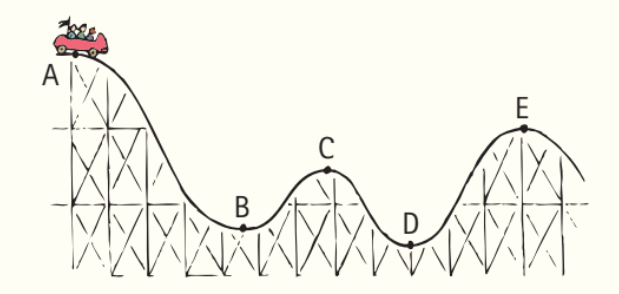### Solution Found!# The roller coaster ride starts from rest at point A. Rank

Chapter , Problem 53TAR

(choose chapter or problem)

Get Unlimited Answers! Check out our subscriptions
QUESTION:

The roller coaster ride starts from rest at point $$A$$. Rank these quantities from greatest to least at each point:

a. Speed

b. $$KE$$

c. $$PE$$Equation Transcription:Text Transcription:

A

KE

PE

QUESTION:

The roller coaster ride starts from rest at point $$A$$. Rank these quantities from greatest to least at each point:

a. Speed

b. $$KE$$

c. $$PE$$Equation Transcription:Text Transcription:

A

KE

PE

Step 1 of 2

According to energy conservation, the highest point from the bottom will have the highest potential energy and lowest kinetic energy. The potential energy depends on the object's height, and the kinetic energy depends on the object's speed.

When the car starts from point A, it has the maximum potential energy and minimum kinetic energy. The potential energy reduces as the car moves towards point B, and kinetic energy increases. After point B, the potential energy increases due to the increase in height, and the kinetic energy decreases until point C. For path C-D, the potential energy decreases and kinetic energy increases, and the same process proceeds for point E.